# Matplotlib 绘图快速入门2021年4月10日07:20:47

2 5911字阅读19分42秒

## 简介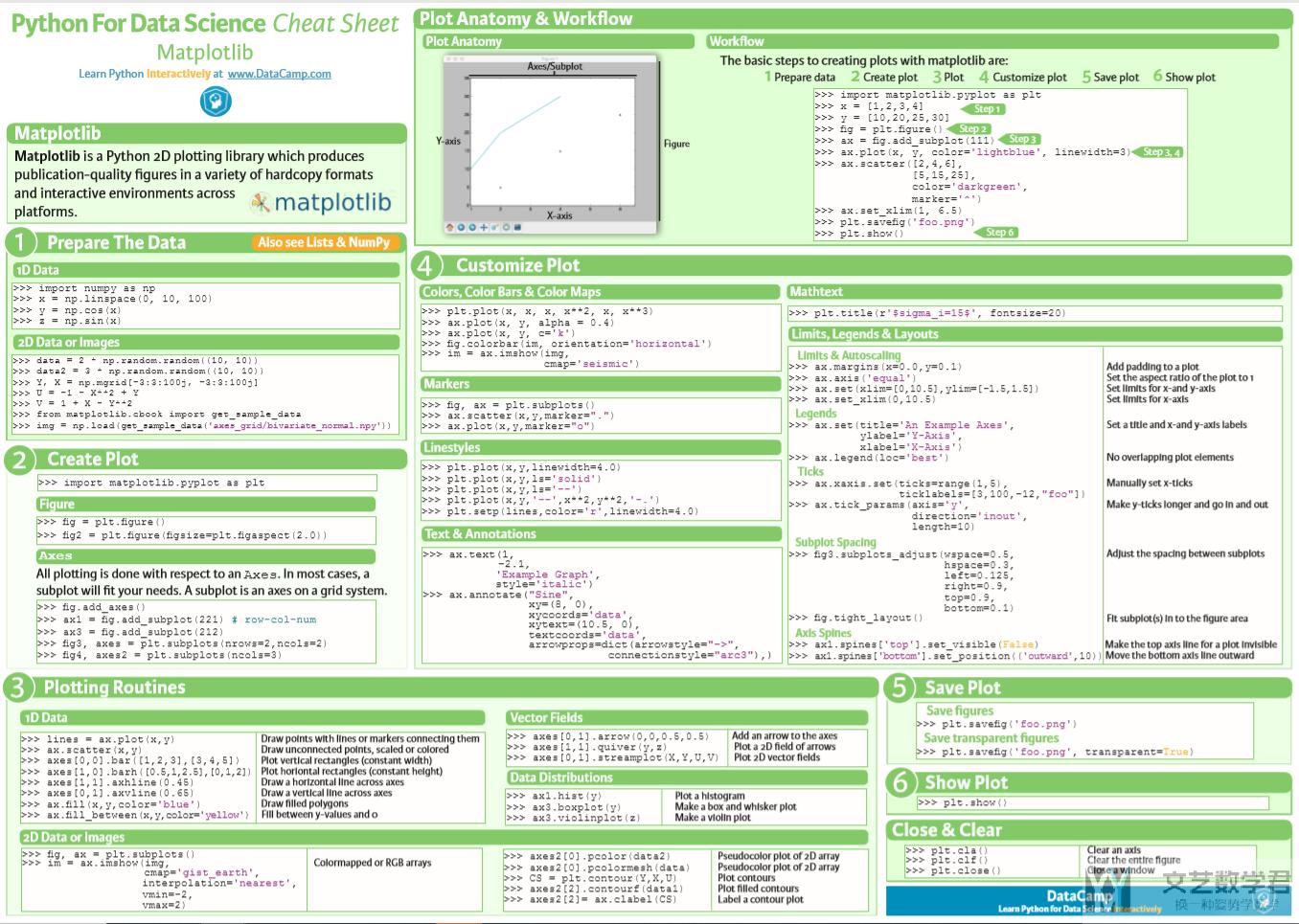## Matplotlib 绘图快速入门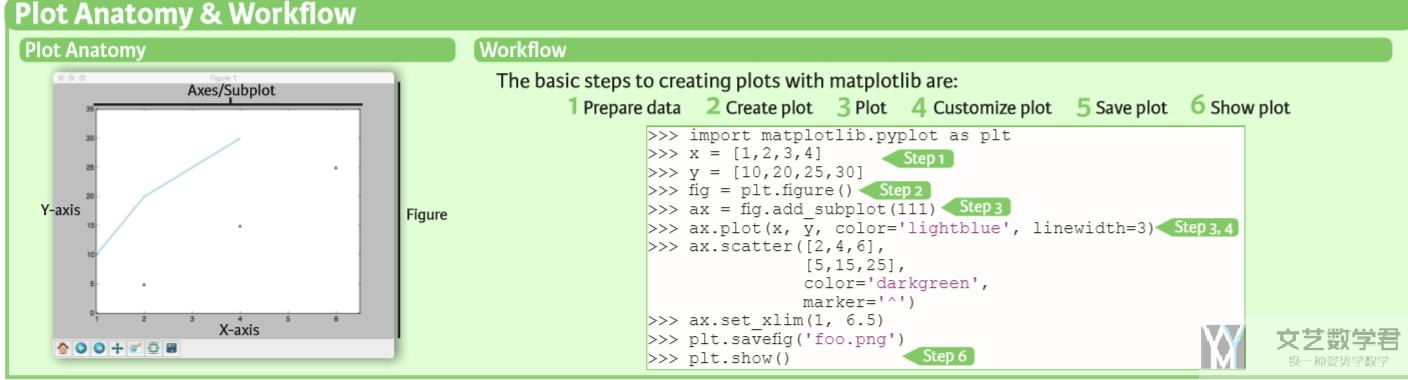### 数据集准备

1. import numpy as np
2. x = np.linspace(0, 10, 100)
3. y = np.cos(x)
4. z = np.sin(x)

1. # 方法一
2. data = 2*np.random.random((10, 10)) # 创建 10*10 大小的数据
3. # 方法二
4. Y, X = np.mgrid[-3:3:100j, -3:3:100j]
5. U = -1 - X**2 + Y
6. V = 1 + X - Y**2

### 创建绘图-create plot

1. import matplotlib.pyplot as plt

1. fig = plt.figure()
2. fig2 = plt.figure(figsize=plt.figaspect(2.0)) # width and height 比例

创建 Axes。每一个的绘图都是与 Axes 有关的。一个 subplot 对应一个 Axes。会有以下两种方式添加 subplot，第一种是依次进行添加，第二种可以一下指定大小（这里推荐使用方法二）。

1. # 方法一
3. ax1 = fig.add_subplot(221) # row-col-num
5. # 方法二
6. fig3, axes = plt.subplots(nrows=2,ncols=2)
7. fig4, axes2 = plt.subplots(ncols=3)

### 开始绘图-基础图像

1. fig = plt.figure(figsize=plt.figaspect(0.3)) # step-2
2. ax1 = fig.add_subplot(131) # row-col-num
5. ax1.scatter(x, y) # step-3
6. ax2.fill(x, y, color='blue')
7. ax3.fill_between(x, y, color='yellow') # y 与 y=0 直接进行填充
8. plt.show() # step-6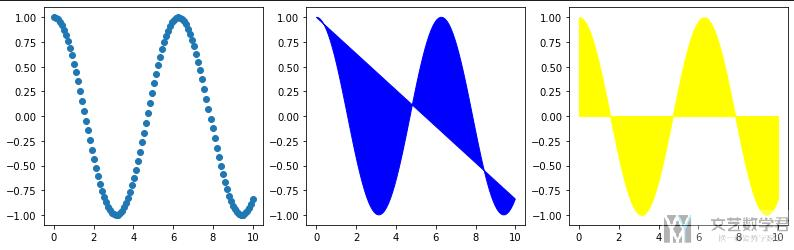1. fig, axes = plt.subplots(figsize=(8, 8), nrows=2,ncols=2)
2. axes[0, 0].bar([1,2,3],[3,4,5]) # 柱状图
3. axes[1, 0].barh([0.5, 1, 2.5],[0, 1, 2]) # 横向柱状图
4. axes[1, 1].axhline(0.45) # 绘制与 x 轴平行的线
5. axes[0, 1].axvline(0.65) # 绘制与 y 轴平行的线
6. plt.show()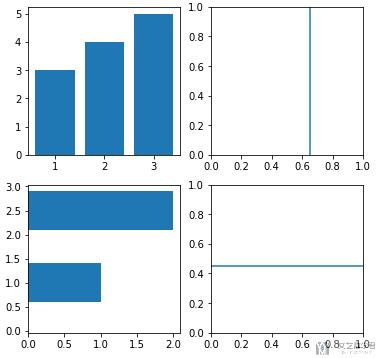#### 一维数据基础图像

1. fig = plt.figure(figsize=plt.figaspect(0.3))
5. ax1.hist(y)
6. ax2.boxplot(y)
7. ax3.violinplot(y)
8. plt.show()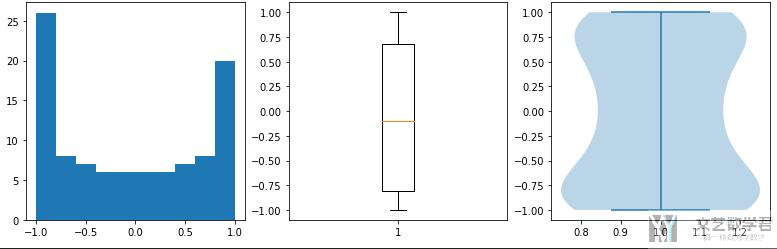#### 二维数据基础图像

1. fig, axes = plt.subplots(figsize=(7, 7), nrows=2,ncols=2)
2. axes[0, 0].pcolor(data)
3. axes[1, 0].pcolormesh(data)
4. axes[0, 1].contour(data)
5. axes[1, 1].contourf(data) # 绘制等高线
6. plt.show()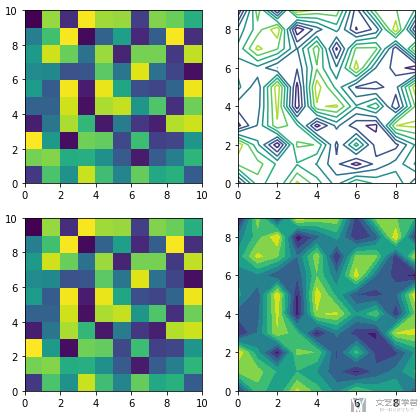### 自定义绘图的样式

#### 定制标题

1. fig = plt.figure(figsize=plt.figaspect(0.5))
4. ax1.plot(x, y, linestyle='--') # 定制线条样式
5. ax1.set_title(r'\$Cos(x)\$', fontsize=15)
6. ax2.scatter(x, z)
7. ax2.set_title(r'\$Sin(x)\$', fontsize=15)
8. plt.show()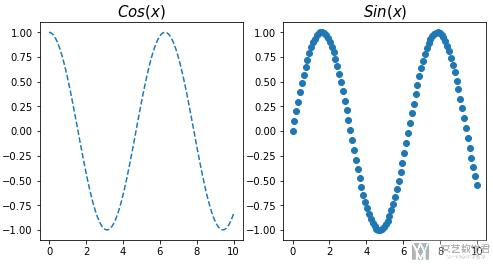#### 设置图例（label and legend）

1. fig = plt.figure(figsize=plt.figaspect(0.5))
4. ax1.plot(x, y, linestyle='--', label=r'\$cos\$')
7. ax1.legend()
8. ax2.scatter(x, z)
9. plt.show()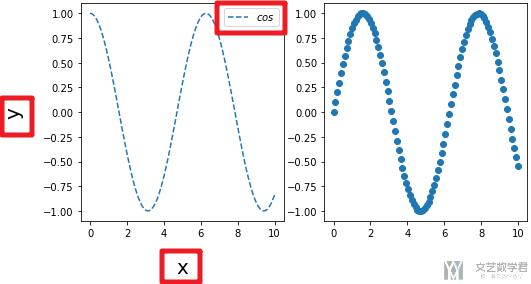#### 设置 x 和 y 轴的范围（limits）

1. fig = plt.figure(figsize=plt.figaspect(0.5))
4. ax1.plot(x, y, linestyle='--')
5. ax1.margins(x=2, y=1) # 控制边距
6. ax2.scatter(x, z)
7. ax2.set(xlim=[0, 10], ylim=[-2, 2]) # 限制 x 和 y 轴的范围
8. plt.show()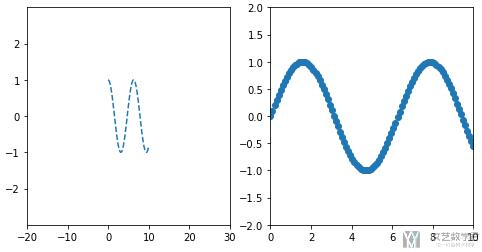#### 设置 Ticks 的内容和大小

1. fig = plt.figure(figsize=plt.figaspect(0.5))
4. ax1.plot(x, y, linestyle='--')
5. ax1.xaxis.set(ticks=range(1,10,2), ticklabels=['a', 'b', 'c', 'd', 'e']) # 自定义 ticks 的内容
6. ax1.xaxis.set_tick_params(labelsize=20, rotation=-45) # 定义大小和旋转
7. ax2.scatter(x, z)
8. plt.show()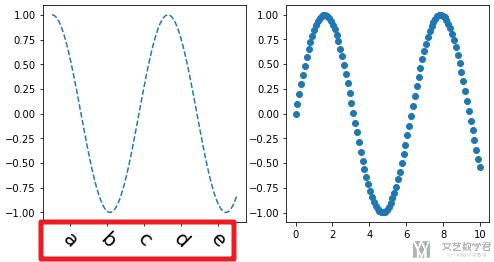#### 设置边框距离与是否显示

1. fig = plt.figure(figsize=plt.figaspect(0.5))
4. ax1.plot(x, y, linestyle='--')
5. ax1.spines['top'].set_visible(False)
6. ax1.spines['left'].set_visible(False)
7. ax1.spines['bottom'].set_position(('outward', 100))
8. ax2.scatter(x, z)
9. plt.show()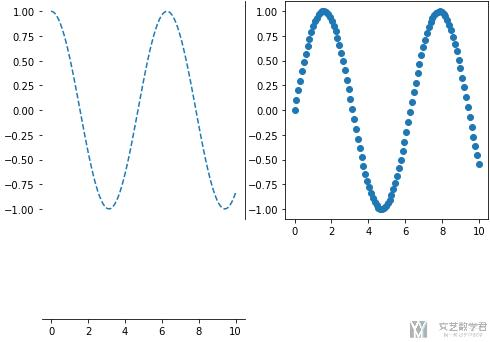#### 子图间的距离

1. fig, axes = plt.subplots(figsize=(7, 7), nrows=2,ncols=2)
3.                    hspace=0.5, # 控制纵向的间距
4.                    left=0.2,
5.                    right=0.9,
6.                    top=0.9,
7.                    bottom=0.1
8.                    )
9. axes[0, 0].pcolor(data)
10. axes[1, 0].pcolormesh(data)
11. axes[0, 1].contour(data)
12. axes[1, 1].contourf(data) # 绘制等高线
13. plt.show()

1. fig.tight_layout()

#### 指定位置显示文本和注解

1. fig = plt.figure(figsize=plt.figaspect(0.5))
4. ax1.plot(x, y, linestyle='--')
5. ax1.text(5, 0.5, 'Example', style='italic', fontsize=20) # 在 (5, 0.5) 处显示内容
6. ax1.annotate("Sine", xy=(6, 1), xycoords='data',
7.             xytext=(1, 0), textcoords='data',
8.             arrowprops=dict(arrowstyle='->', connectionstyle='arc3')) # 注解
9. ax2.scatter(x, z)
10. plt.show()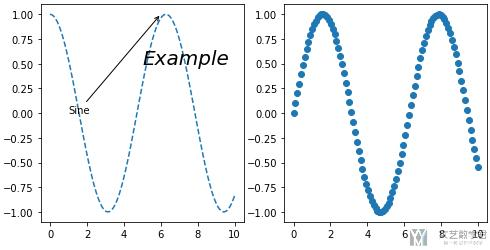#### 定制不同的 Markers

1. fig = plt.figure(figsize=plt.figaspect(0.5))
4. ax1.plot(x, y, marker='.', markersize=9, markerfacecolor='red')
5. ax2.scatter(x, y, marker='o')
6. plt.show()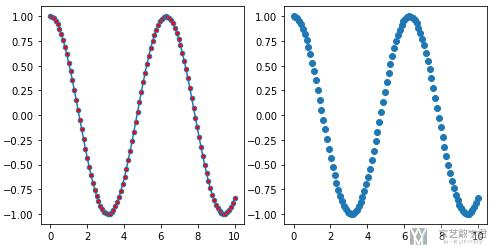#### 定制颜色（color）

1. fig = plt.figure(figsize=plt.figaspect(0.5))
4. ax1.plot(x, y, color='red') # 定制颜色
5. ax2.plot(x, y, color='red', alpha=0.4) # 定制透明度
6. plt.show()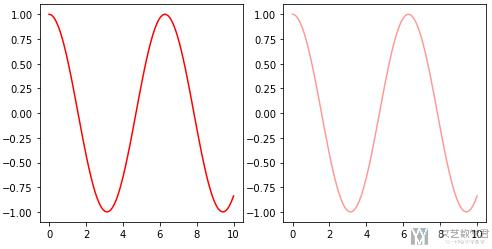#### 定制线条风格（linestyles）

1. fig = plt.figure(figsize=plt.figaspect(0.5))
4. ax1.plot(x, y, linestyle='--') # 定制线条样式
5. ax2.plot(x, y, linewidth=4) # 定制线条宽度
6. plt.show()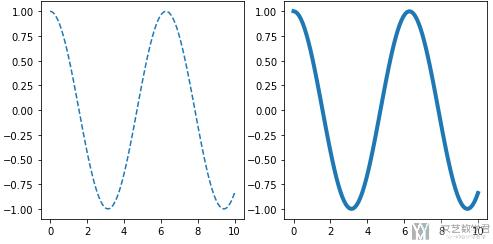### 保存图像

1. # save figures
2. plt.savefig('foo.png')
3. # save transparents figures
4. plt.savefig('foo.png', transparents=True)

### 展示图像

1. plt.show()

1. plt.cla() # clear an axis
2. plt.clf() # clear the entire figure
3. plt.close() # close a windows

• 微信公众号
• 关注微信公众号
•• QQ群
• 我们的QQ群号
•• 本文由 发表于 2021年4月10日07:20:47
• 转载请务必保留本文链接：https://mathpretty.com/13603.html